[简答题]

《概率论》 由 提供  共 43 道[简答题] 投掷两个均匀的骰子，已知点数之和是偶数，则点数之和为6的概率为_____。

[简答题] 将一枚硬币均匀投掷三次，则三次中恰好出现两次正面向上的概率为____。

[简答题] 已知A,B,C两两独立，P(A)=P(B)=P(C)=1/2，P(ABC)=1/5，则 P(ABC)等于_____。

[填空题]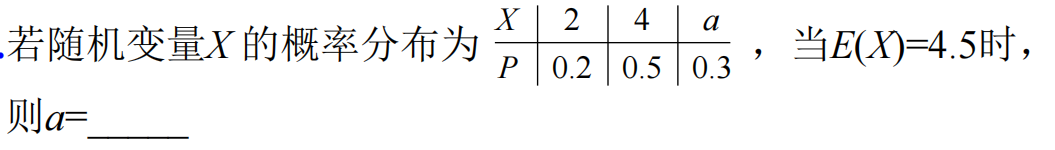[简答题] 掷一枚质地均匀的骰子，则在出现奇数点的条件下出现3点的概率为_____。

[简答题] 设X~N(3,22) ，那么当P(X≤C ) = P(X>C )时，则C=____。

[简答题] 设事件A,B相互独立，P(A)=0.5,P(B)=0.7，则P(A∪B)=_____。

[简答题] 设15只同类型的零件中有2只次品，在其中取3次，每次任取1只，做不放回抽样，则取出的次品数为1的概率为______。

[简答题]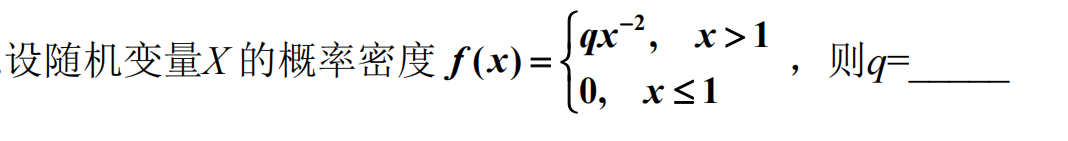[简答题]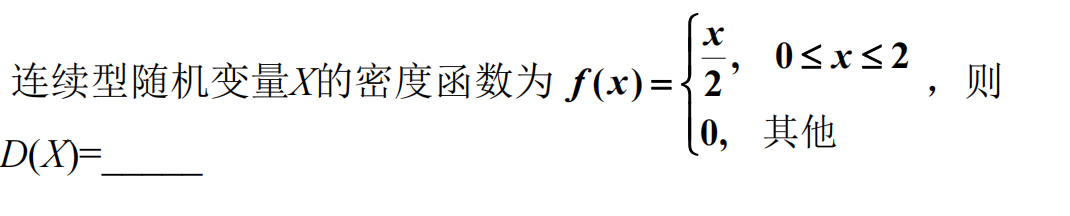[简答题] 设随机事件A, B,C ，则用A, B, C的运算关系表示事件A发生、B与C不发生为_____。

[填空题] 设随机变量X～B(n,p)，且E(X)=2.4，D(X)=1.44，则参数

n=_____，p=_____

[简答题]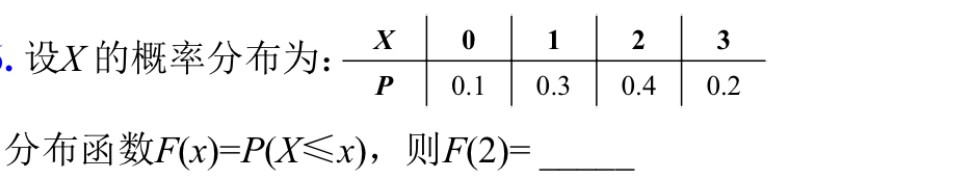[填空题]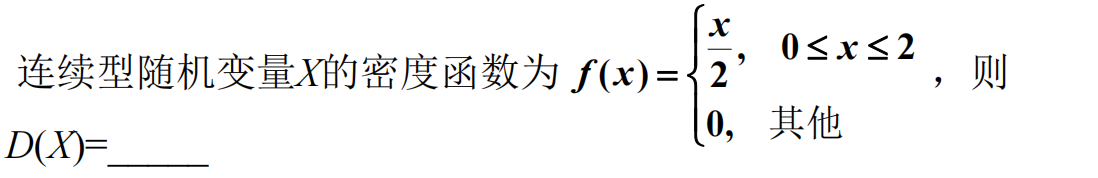[简答题] 某果园生产红富士苹果，一级品率为0.6，随机取10个，恰有 6个一级品之概率为_______。

[简答题] 设X～N(0,1)，X 的分布函数为Ф(x)，则P{|X|>2}=__________

[简答题] 设某小区60%的居民订报纸A，45%的订报纸B，30%的两报均订，随机抽一户，则至少订一种报的概率为 _____。

[填空题] 设箱中有3个红球和2个白球，从中随机取出一球，若是红球

得5分，白球得2分，则所得分数的数学期望为_____

[简答题] 用事件A,B,C表示“A,B,C恰有一个发生”为

[单选题] 设随机事件A,B，且B⊂A，则下列式子正确的是( )

A、

P(A∪B)=P(A)

B、

P(AB)=P(A)

C、

P(B|A)=P(B)

D、

P(B-A)=P(B)-P(A)